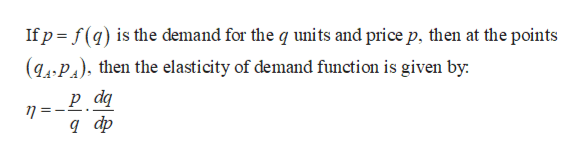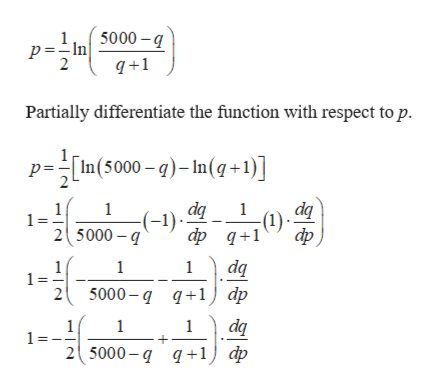Suppose that the demand for a product is given by p= 1/2ln (5000-q/q+1)where p is in hundreds of dollars and q is the number of tons. (a) What is the elasticity of demand when the quantity demanded is 2 tons and the price is \$371?(b) Is the demand elastic or inelastic?

Question

Suppose that the demand for a product is given by

p= 1/2ln (5000-q/q+1)

where p is in hundreds of dollars and q is the number of tons.

(a) What is the elasticity of demand when the quantity demanded is 2 tons and the price is \$371?

(b) Is the demand elastic or inelastic?

Step 1

The elasticity of demand when the quantity demanded (q) is 2 and the price (p) is 3.71 hundreds of dollars.

Step 2

Formula used:help_outlineImage TranscriptioncloseIf p f(q) is the demand for the q units and price p, then at the points (gA.P) then the elasticity of demand function is given by р dg n=- д ф fullscreen
Step 3

The given funct...help_outlineImage Transcriptionclosep =In 3000-g 2 q+1 Partially differentiate the function with respect to p. p=In(5000-)-In(q+1)] dq dq 1 1 (1) фф д+1 (-1) 1 25000 фр, dq 1 1 1 = 2 5000-д д+1) dp da 2( 5000- q q+1) ф 1 1 fullscreen

Want to see the full answer?

See Solution

Want to see this answer and more?

Our solutions are written by experts, many with advanced degrees, and available 24/7

See Solution
Tagged in

Other